# MCQs & Assertion Reason Haloalkanes and Haloarenes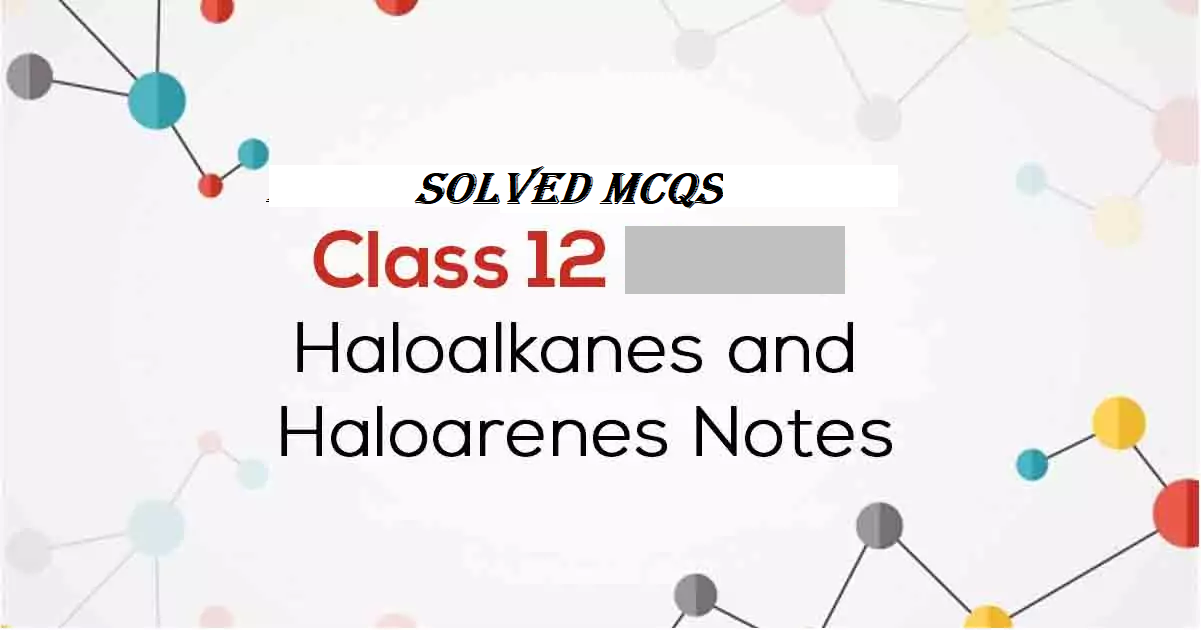MCQs & Assertion Reason Haloalkanes and Haloarenes

MCQs & Assertion Reason Haloalkanes and Haloarenes contain important questions of Haloalkanes and Haloarenes

Q 1. Arrange the following compounds in increasing order of rate of reaction towards nucleophilic substitution: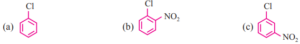(a) a < b < c                                         (b) a < b < a
(c) a < c < b                                         (d) c < a < b

Q 2. Arrange the following compound in increasing order of rate of reaction towards nucleophilic substitution.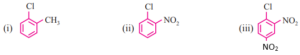(a) i < ii < iii                                         (b) i < iii < ii
(c) ii < i < iii                                         (d) iii < ii < i

Q 3. Which of the following compound will undergo racemization when the solution of KOH hydrolyses?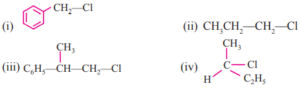(a) i and iv                                           (b) ii and iv
(c) iii and iv                                         (d) iv

Q 4. In a SN1 reaction on chiral centres, there is
(a) 100 % retention
(b) 100 % inversion
(c) 100 % racemisation
(d) inversion is more than retention leading to racemisation

Q 5. The reaction of C6H5—CH=CH—CH3 with HBr produces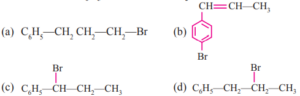MCQs & Assertion Reason Haloalkanes and Haloarenes

Q 6. In SN2 reactions, the correct order of reactivity for the following compounds:
(i) CH3Cl
(ii) (CH3)3CCl
(iii) (CH3)2CHCl
(iv) CH3CH2—Cl
(a) i > ii > iii > iv                                 (b) iv > iii > ii > i
(c) i > iv > iii > ii                                 (d) iv > i > ii > iii

Q 7. Arrange the following compounds in increasing order of their boiling point:
(i) (CH3)2CH2CH2—Br
(ii) CH3—(CH2)—Br3
(iii) (CH3)3C—Br
(a) ii < i < iii                                       (b) i < ii < iii
(c) iii < i < ii                                       (d) iii < ii < i

Q 8. Toluene reacts with halogen in the presence of FeCl3 giving ortho and para compounds. The reactions is:
(a) electrophilic elimination reaction                          (b) electrophilic substitution

Q 9. In the reaction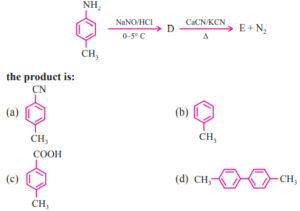Q10. Chlorobenzene is formed by the reaction of chlorine with benzene in presence of AlCl3. Which of the following species attacks the benzene ring in this reaction?
(a) Cl                                                               (b) Cl+
(c) AlCl3                                                             (d) AlCl4

MCQs & Assertion Reason Haloalkanes and Haloarenes

Q11. The reaction of toluene with Cl2 in the presence of FeCl3 gives ‘X’ and the reaction with Cl2 in presence of light gives ‘Y’. Thus ‘X’ and ‘Y’ are:
(a) ‘X’ = benzyl chloride and ‘Y’ = m-chlorotoluene
(b) ‘X’ = benzyl chloride and ‘Y’ = o-chlorotoluene
(c) ‘X’ = m-chlorotoluene and ‘Y’ = p-cholorotoluene
(d) ‘X’ = p-chlorotoluene and ‘Y’ = benzyl chloride.

Q12. Aryl halides are less reactive toward nucleophilic substitution reaction than alkyl halides due to
(a) the formation of stable carbanion
(b) resonance stabilization
(c) longer carbon-halogen bond
(d) sphybridised carbon attached to the halogen

Q13. A new carbon carbon bond is possible in the following reaction reactions:
(a) C6H6 + CH3Cl3 → (anhy. AlCl3)
(b) CH3CH2Br + CH3CH2NH2
(c) CH3 —Br + CH3CH2—ONa →
(d) CH3CH2—Br + kCN(alc) →

Q 14. Which of the following state are correct
(a) Benzyl halides are more reactive than vinyl and aryl halides
(b) Vinyl/halides are more reactive than alkyl halides
(c) Aryl halides are less reactive than alkyl halide
(d) Aryl halides are more reactive than benzyl halides

MCQs & Assertion Reason Haloalkanes and Haloarenes

Note: In the following questions a statement of assertion followed by a statement of reason is given. Choose the correct answer out of the following choice.
(a) Both assertion and reason are correct, and the reason is the correct explanation of the assertion.
(b) Both assertion and reason are true, but the reason is not the correct explanation of the assertion.
(c) Assertion is Incorrect, but the reason is Correct.
(d) Both assertion and reason are Incorrect.

Q 15. Assertion: SN2 reaction proceeds with inversion of configuration.
Reason: SN2 reaction occurs in one step

Q 16. Assertion: Treatment of chloroethane with a saturated solution of AgCN gives ethyl isocyanide as a major product.
Reason: Cyanide ion (CN) is an ambident nucleophile.

MCQs & Assertion Reason Haloalkanes and Haloarenes

Matching Column Type
Q 17. Match the items of column 1 and column 2
Column 1                                                            Column 2
(A) CH2Cl2                                                      P. Antiseptic
(B) CCl4                                                           Q. Insecticide
(C) (p-ClC6H4)CHCCl3                                  R. Pyrene
(D) CHI3                                                           S. Refrigerant
(a) A–R, B–Q, C–S, D–P
(b) A–S, B–R, C–Q, D–P
(c) A–Q, B–P, C–S, D–R
(d) A–P, B–S, C–R, D–Q

Q18. Match the items of column 1 and column 2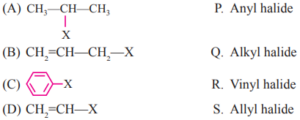(a) A–P, B–Q, C–S, D–P                                                  (b) A–S, B–R, C–Q, D–P
(c) A–Q, B–P, C–S, D–R                                                  (d) A–P, B–S, C–R, D–Q

The Answers of MCQs & Assertion Reason Haloalkanes and Haloarenes are given below:
Q1. (c)     Q2.(a)       Q3. (d)    Q4. (d)     Q5.(c)     Q6. (c)      Q7. (c)

Q8. (b)     Q9. (a)      Q10. (b)   Q11.(d)    Q12.(b, d) Q13.(a, d)   Q15. (a, c)

Q 16. (b)   Q17. (b)      Q18.(b)

MCQs & Assertion Reason Haloalkanes and Haloarenes